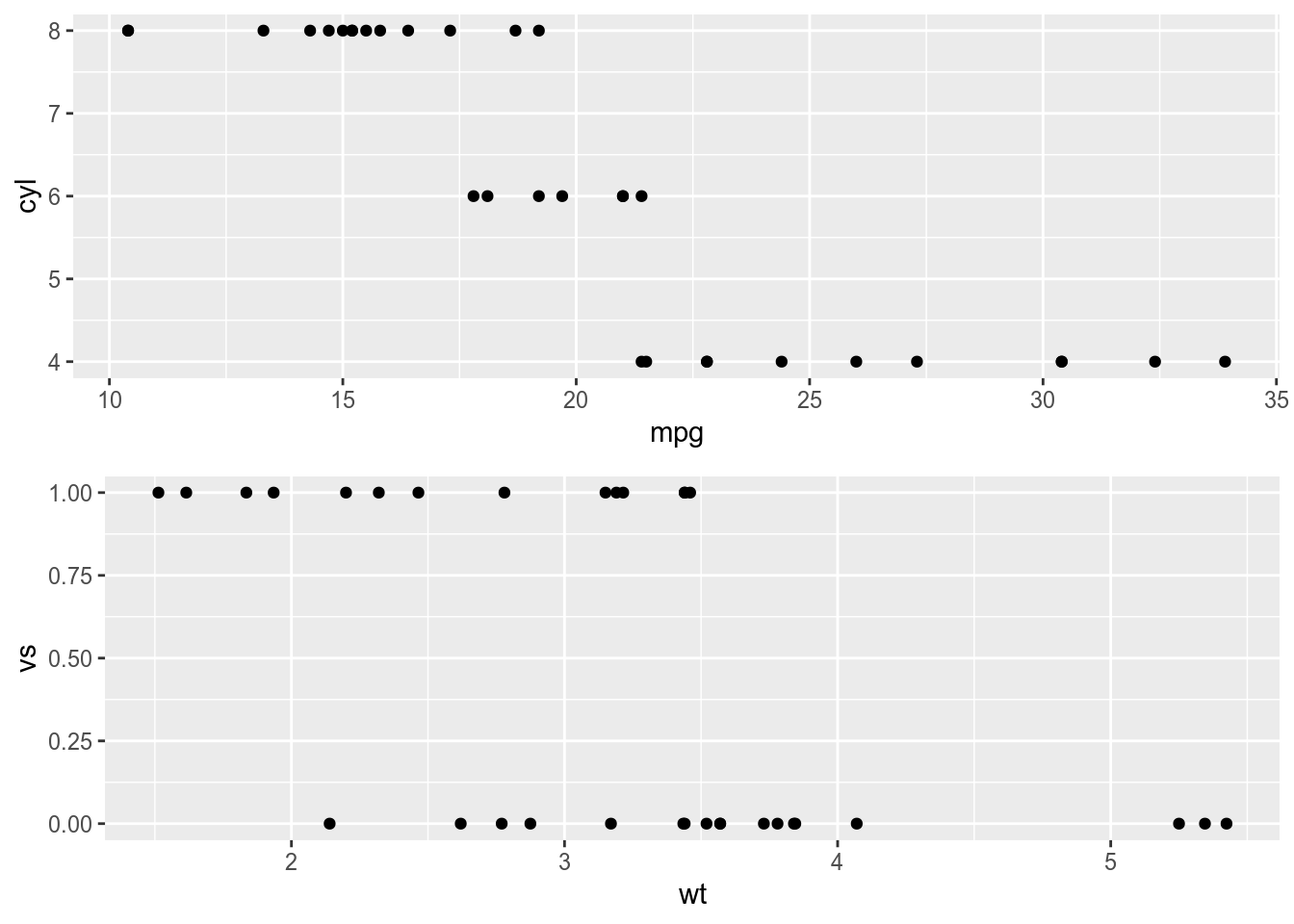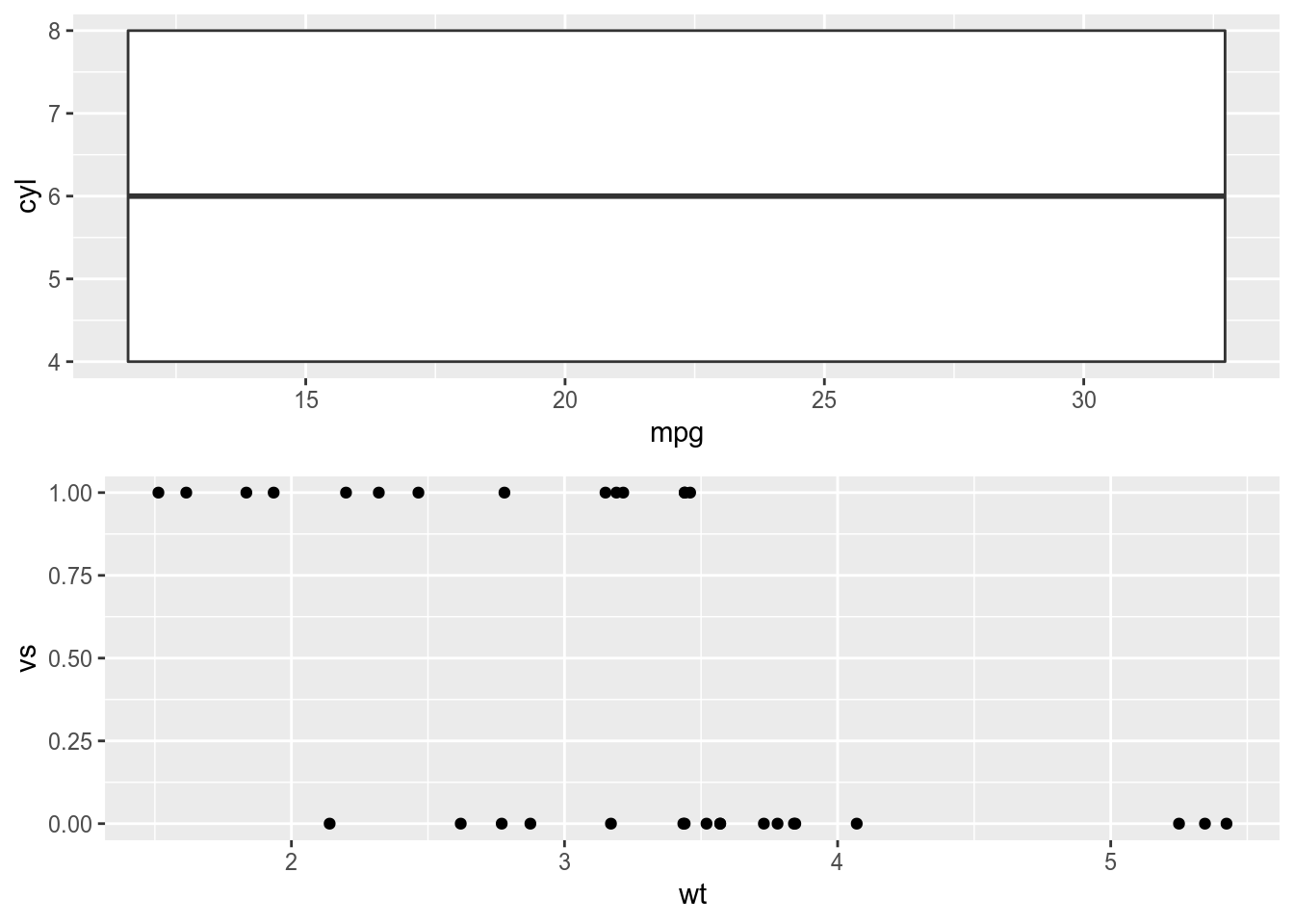## R Markdown

test for cache dependencies

# 1st time a:
# gg1 = ggplot(mtcars, aes(x= mpg, y= cyl)) +geom_point()

# 2nd time, gg1 changed
gg1 = ggplot(mtcars, aes(x= mpg, y= cyl)) +geom_boxplot()

gg2 = ggplot(mtcars, aes(x= wt, y= vs)) +geom_point()


### chunk2, no depends, cached what’s left in 1st run

grid.arrange(gg1,gg2)### chunk3, depends on chunk1,

grid.arrange(gg1,gg2)
## Warning: Continuous x aesthetic -- did you forget aes(group=...)?